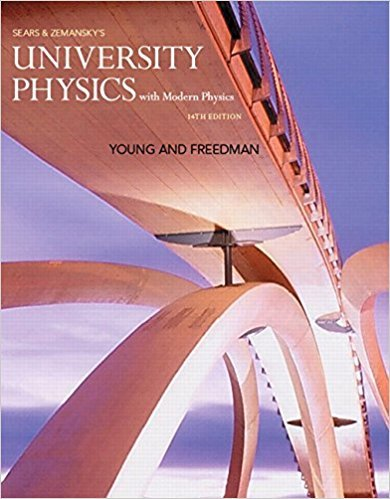# Solution: A cat walks in a straight line, which we shall## Problem 2.3 Chapter 2

University Physics with Modern Physics (1) | 14th Edition

• 2901 Step-by-step solutions solved by professors and subject experts
• Get 24/7 help from StudySoup virtual teaching assistantsUniversity Physics with Modern Physics (1) | 14th Edition

4 5 0 357 Reviews
27
5
Problem 2.3

A cat walks in a straight line, which we shall call the x-axis, with the positive direction to the right. As an observant physicist, you make measurements of this cats motion and construct a graph of the felines velocity as a function of time (Fig. E2.30). (a) Find the cats velocity at t = 4.0 s and at t = 7.0 s. (b) What is the cats acceleration at t = 3.0 s? At t = 6.0 s? At t = 7.0 s? (c) What distance does the cat move during the first 4.5 s? From t = 0 to t = 7.5 s? (d) Assuming that the cat started at the origin, sketch clear graphs of the cats acceleration and position as functions of time.

Step-by-Step Solution:
Step 1 of 3

Chapter 2 Notes When mass is at an equilibrium and the spring is stretched in equilibrium the force of gravity balances the elastic force from a spring. The elastic force depends on the elongation of our string F= -kx Where x is elongation and k is the stiffness or spring constant. K depends on thickness of the wire as well as on the material our spring is made of. Please note the...

Step 2 of 3

Step 3 of 3

##### ISBN: 9780321973610

The full step-by-step solution to problem: 2.3 from chapter: 2 was answered by Patricia, our top Physics solution expert on 01/09/18, 07:46PM. The answer to “A cat walks in a straight line, which we shall call the x-axis, with the positive direction to the right. As an observant physicist, you make measurements of this cats motion and construct a graph of the felines velocity as a function of time (Fig. E2.30). (a) Find the cats velocity at t = 4.0 s and at t = 7.0 s. (b) What is the cats acceleration at t = 3.0 s? At t = 6.0 s? At t = 7.0 s? (c) What distance does the cat move during the first 4.5 s? From t = 0 to t = 7.5 s? (d) Assuming that the cat started at the origin, sketch clear graphs of the cats acceleration and position as functions of time.” is broken down into a number of easy to follow steps, and 126 words. Since the solution to 2.3 from 2 chapter was answered, more than 219 students have viewed the full step-by-step answer. University Physics with Modern Physics (1) was written by Patricia and is associated to the ISBN: 9780321973610. This textbook survival guide was created for the textbook: University Physics with Modern Physics (1), edition: 14. This full solution covers the following key subjects: . This expansive textbook survival guide covers 44 chapters, and 4574 solutions.

×
Get Full Access to University Physics With Modern Physics (1) - 14 Edition - Chapter 2 - Problem 2.3

Get Full Access to University Physics With Modern Physics (1) - 14 Edition - Chapter 2 - Problem 2.3

I don't want to reset my password

Need help? Contact support

Need an Account? Is not associated with an account
We're here to help